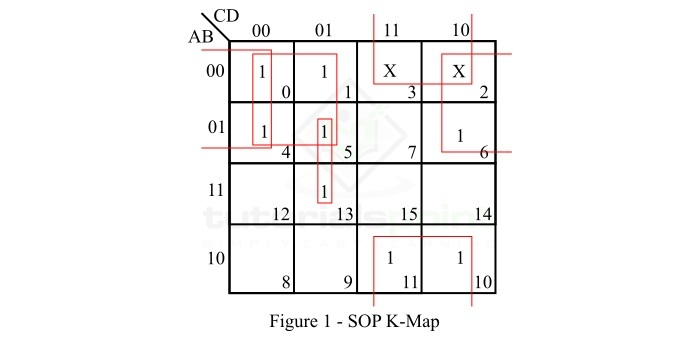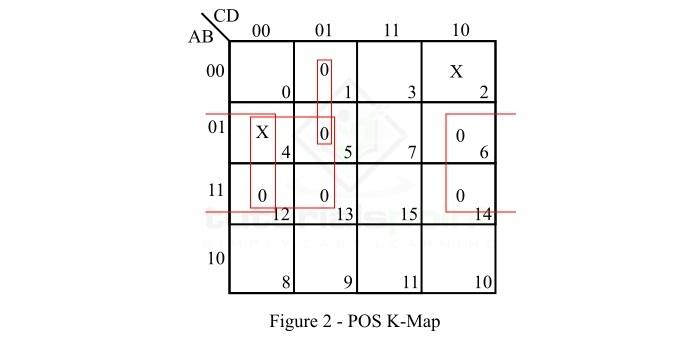# Don't Care (X) Conditions in K-Maps

K-Map or Karnaugh Map is a graphical method of simplifying Boolean expression. A K-Map composed of an arrangement of adjacent squares or cells, where each cell represent a particular combination of variables in sum or product form.

In the K-map method, there is a useful condition namely, Don’t Care Condition, which helps in simplifying a Boolean function. The don’t care condition makes the grouping of variables in K-map easy. In this tutorial, we will understand the "don’t care" concept in K-map reduction with the help of solved examples.

Sometimes, in a Boolean expression for certain input combinations, the value of the output is not specified either due to invalid input combinations or due to the precise value of output is of no importance. These input combinations for which the values of the Boolean function are not specified are called don’t care combinations. Don’t care combinations are also referred to as optional combinations. In K-map, don’t care combinations are represented by "X" or "d" or "ϕ".

For example, in 8421 binary code, the binary combination 1010, 1011, 1100, 1101, 1110, and 1111 are the invalid terms and their corresponding outputs are don’t care combinations. Similarly, in Excess-3 code, the combinations 0000, 0001, 0010, 1101, 1110, and 1111 do not occur, hence these are called don’t care combinations.

When we deal with SOP (Sum of Products) K-map, each don’t care term is treated as a 1, if it helps in reduction of the expression, otherwise it is considered as a 0 and left alone.

On the other hand, when we are using POS (Product of Sums) K-map, each don’t care term is considered as a 0, if it is helpful in reduction of the expression, otherwise it is treated as a 1 and left alone.

Also, we can convert a Standard Sum of Products (SSOP) expression with don’t care terms into a Standard Product of Sums (SPOS) expression by keeping the don’t care terms as they are, and writing the missing minterms of the SSOP form as the maxterms of the SPOS form.

Similarly, we can convert a standard product of sums (SPOS) expression with don’t care terms into a standard sum of products (SSOP) expression by keeping the don’t care terms of the SPOS expression as they are, and writing the missing maxterms of the SPOS expression as the minterms of the SSOP expression.

Now, let us discuss some solved examples to understand the don’t care condition in K-map.

## Example 1

Minimize the following 4-variable Boolean expression in SOP form using K-map.

$$\mathrm{\mathit{f}\lgroup A,B,C,D\rgroup=\sum m \lgroup 0,1,4,5,6,10,13\rgroup +d\lgroup 2,3\rgroup}$$

### Solution

The SOP K-map representation of the given Boolean function is shown in Figure 1.As we can see in the given expression, there are two don’t care terms which are denoted by X on the K-map.

### Explanation

The reduction of the expression is done as per the following steps −

• The minterms m0, m1, m4, and m5 form a 4-square. Make it and read it as $\lgroup\overline{A}\:\overline{C}\rgroup$.

• The minterms m10 and m11 form a 4-sqaure with two don’t care terms m2 and m3. Make it and read it as $\overline{B}C$.

• The minterms m0, m4, m6, and the don’t care term m2 together form a 4-square. Make it and read it as $\overline{A}\:\overline{D}$.

• The minterms m5 and m13 form a 2-square. Make it and read it as $B\overline{C}D$.

• Finally, write all the product terms in SOP form.

Therefore, the minimal Boolean expression is,

$$\mathrm{\mathit{f}\lgroup A,B,C,D\rgroup=\overline{A}\:\overline{C}+\overline{B}C+\overline{A}\:\overline{D}+B\overline{C}D}$$

## Example 2

Minimize the following 4-variable Boolean expression in POS form using K map.

$$\mathrm{\mathit{f}\lgroup A,B,C,D\rgroup=\prod M\lgroup 1,5,6,12,13,14\rgroup+d\lgroup 2,4\rgroup}$$

### Solution

The POS K-map representation of the given Boolean function is shown in Figure-2.There are two don’t care terms in the expression which are represented by X on the K-map.

### Explanation

The reduction of the given function is done as per the following steps −

• The maxterms M5, M12, and M13 and don’t care term M4 form a 4-square. Make it and read it as $\overline{B}+C$.

• The maxterms M6, M12, and M14 and don’t care term M4 form a 4-square. Make it and read it as $\overline{B}+D$.

• The maxterms M1 and M5 form a 2-square. Make it and read it as $A+C+\overline{D}$

• Write all the sum terms in POS form.

Therefore, the minimal Boolean expression is,

$$\mathrm{\mathit{f}\lgroup A,B,C,D\rgroup=\lgroup\overline{B}+C\rgroup+\lgroup\overline{B}+D\rgroup+\lgroup A+C+\overline{D}\rgroup}$$

## Conclusion

This is all about don’t care conditions in K-maps. As we discussed in the above sections of this tutorial, that the don’t care condition is a significant and powerful concept that helps in minimization of Boolean function using K-map.

Updated on: 03-Oct-2023

734 Views